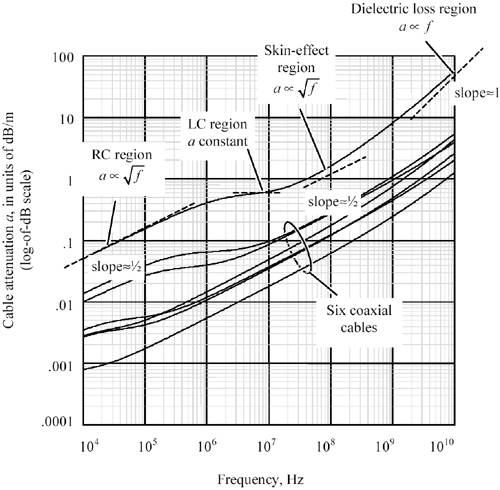# Performance Regions

Figure 3.1 displays the propagation function of six distinct types of coaxial cabling, plus one typical pcb trace. The horizontal axis shows the operating frequency in units of Hz. The vertical axis shows cable attenuation in units of dB. Both axes use logarithmic scales . 

 Because the decibel is already a logarithmic unit, the vertical axis is actually a double-log (logarithm of a logarithm) of the cable propagation function.

Figure 3.1. The attenuation curve for all copper transmission media is divided into distinct regions, with a characteristic relation in each region between the attenuation a and frequency.Each curve may be divided into distinct regions, with a characteristic shape to the loss function in each region. The hierarchy of regions, in order of increasing frequency, proceeds generally in the same order for all copper media:

• RC region
• LC region
• Skin-effect region
• Dielectric loss region
• Waveguide dispersion region

Within each region the requirements for termination differ , as do the tradeoffs between length and speed. Remarkably, a common signal propagation model accurately describes almost any type of metallic transmission media across all four regions.High-Speed Signal Propagation[c] Advanced Black Magic
ISBN: 013084408X
EAN: N/A
Year: 2005
Pages: 163